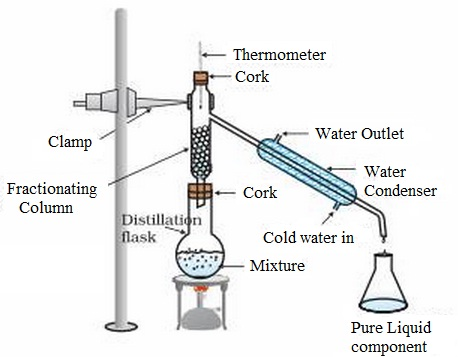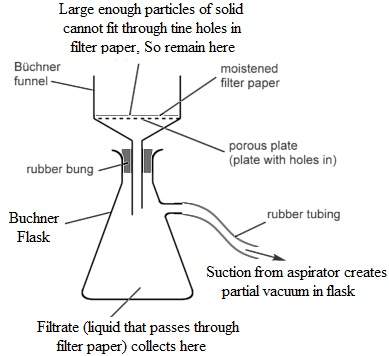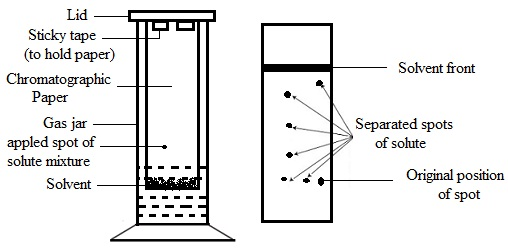#### Purification Methods-Empirical and molecular formula, Chemistry tutorial

Introduction:

The Organic compounds are generally represented in organic chemistry with formula, which is a shorthand method of exhibiting the constituent atoms. The formula of a compound comprises of the chemical symbols of the constituent atoms and numerical subscript to point out the ratio of the atoms. Remember that the chemical bonds hold atoms altogether in the molecule and for organic compounds, the bond is basically covalent. In the molecular orbital theory of covalent bonding, a bond is thought of in terms of the overlapping of two atomic orbitals each having one electron. Covalent bonding formed by overlapping of atomic orbitals is of two kinds, the sigma (σ) bond and the pie (π) bond. Each and every bond in organic compounds are of the σ-type; double bonds comprises of one π-bond and one it bond types; whereas triple bonds comprise of one σ-bond and two π-bond types. However whenever bonds between the atoms are drawn, they are all symbolized by a straight line (-), it is significant to note that these two kinds of bonds exist as they find out the reactivity of the organic compounds.

Once an organic compound is made in the laboratory or isolated from a few natural sources, the method for the determination of its nature comprises of the given steps.

1) State of purity and purification.

2) Determination of the elemental composition-qualitative analysis.

3) Determination of the relative numbers of each and every atom present in one molecule of the compound-quantitative analysis,

4) Determination of the empirical and molecular formula.

5) Finding out the structure of molecule.

Purification Methods:

The investigation of any compound should be preceded through careful purification to separate the pure substance from the impurities. In order to separate any two substances it is essential to find out a few differences between them and the generally employed ones are differences in solubility or vapor pressure (boiling point). The greater is the differences between the two compounds the simpler it is to separate them.

Distillation:

This is a method employed in separating the two or more volatile, miscible liquids having different boiling points. For illustration: a mixture of two liquids A (B.P. = 50°C) and B (B.P. 100°C). Distillation is of two kinds - simple distillation and fractional distillation. The simple distillation is utilized whenever the difference in boiling points, of the compounds to be separated is high; whereas fractional distillation is needed whenever the boiling points are close and some compounds are to be separated.

The method of distillation comprises boiling the mixture in a flask and the vapor from it is passed via a condenser. Pure liquid collects in a receiver and is termed to as the distillate; the impurities remain in the distillation flask. The simple arrangement is as shown below:Fig: Simple distillation

The procedure of fractional distillation is not, though, for all time simple and straightforward as illustrated above. It comprises the use of fractionating column for good separation of liquids with extremely similar boiling points.

Crystallization:

It is the commonest procedure for purifying a solid. The method of crystallization comprises determining a solvent, via experimental trial, in which the solid is more soluble if the solvent is hot than when it is cold.

The impure solid is then dissolved in the minimum quantity of hot solvent; the purpose is to get a solution that is nearly saturated at the boiling point of the solvent. The hot solution is then filtered, via a filter paper or funnel, in such a way that any insoluble impurities can be eliminated. The filtration should be fast to avoid crystallization throughout the process. The Buchner flask and funnel is the most appropriate.The soluble impurities remain, altogether with the solid needed, in the filtrate. This is cooled for crystallization and the crystals formed are filtered off via a Buchner funnel. The crystals are then washed in the funnel by a little of the cold solvent and allowed to dry by pressing between the filter papers or in a desicator or oven. The Crystallization is mainly used in the manufacture of drugs and sugar.

Chromatography:

Most of the natural products, for illustration extract from the plants and animals, are specifically hard to purify. They might only be available in small quantities, they tend to decompose on heating, and the impurities are usually chemically identical to the product of interest. Chromatography is a helpful separation method in situations where traditional methods of separation are not successful. Chromatography includes moving a solvent over a porous, adsorbent medium (example: paper or powdered material like silica and alumina) to separate the mixture of solute. There are numerous kinds of chromatographic methods however our main concern is paper chromatography and column chromatography.

(a) Paper chromatography:

These use one strip of chromatographic or filter paper as the adsorbent medium. The solution having the mixture of solutes to be separated is spotted near one end of the paper. The paper is then suspended or hanged in a closed air-tight jar with the spotted end (however not the spot) dipped to an appropriate solvent example hexane, water. As the solvent front rises up the paper via ascending capillary action the different solutes present are carried forward at different speed.  If the solvent front reaches nearly to the top of the paper, the paper is detached and dried. If the solutes are colored their positions on the paper can be observed. For colourless solutes the paper can be treated by suitable chemical reagent to transform the solutes into colored compounds. Each and every solute can then be recognized through the distance travelled and separated via cutting up each and every spot on the paper and each solute extracted separately. The figure shown below represents a typical ascending paper chromatography set up.Fig: Ascending paper chromatography

(b) Column chromatography

In column chromatography, the solution having the solutes is passed via a glass tube or column packed by solid adsorbent like alumina or silica. The mixture is then washed or moved via the column by using a suitable solvent. The solutes move via the column at different speed and are collected as the separate component or fractions as the solvent elutes the column. The solvent is then evaporated to get each and every component of the mixture.

Most of the different packing materials example: alumina, silica, starch, charcoal, calcium carbonate, can be employed. Typical solvents, which might be mixed altogether, comprise water, propanone, ethanol, benzene, trichloromethane and hexane. Chromatographic method has been successfully employed in the petroleum industry, food industry, hospitals and research organization to effect the separation of mixtures of compounds.

Empirical and Molecular Formula:

The Qualitative and quantitative elemental analysis is employed to know the different elements present and their relative composition correspondingly. We will now illustrate the next phase comprised in the identification of an unknown organic compound, which is structural formula determination. The phases comprising the determination of the empirical formula and molecular formula of the compound first; and then the structure that illustrates the manner the atoms are linked to one other, is then determined.

Empirical formula:

This gives the information regarding the simplest ratio of the different atoms in a molecule of compound; it doesn't provide the actual number of atoms in the molecule. For illustration: the empirical formula of ethane, CH3CH3 is CH3 which is similar for all members of the alkane series. For ethanoic acid, CH3CO2H, the empirical formula is CH20 which is similar for glucose, C6H12O6. The empirical formula, thus, provides the simple relative ratio of atoms of each and every element present in the molecule of the compound.

The fundamental steps comprised in the determination of the empirical formula of an organic compound are as follows:

1) Write down the percentage by mass of each element in the compound and the sum should add up to 100% (ignore experimental error). As a rule, if the sum is less than 100 percent, then the difference is allocated to the oxygen atom.

2) The percentage composition of each and every element is divided through the relative atomic mass of the elements in order to get the ratio of the moles of atoms present.

3) Then divide each and every ratio by the smallest ratio value to get the simplest whole number ratio (that is, empirical ratio).

Molecular Formula:

The molecular formula of a compound provides the exact number of moles of atoms of the component elements in one mole of the compound. In certain compounds, the empirical formula and the molecular formula are similar. In other cases, the molecular formula is a simple multiple, 'n', of the empirical formula. For example - ethanol, C2H6O consists of similar empirical formula and molecular formula; whereas both ethyne C2H2 and benzene C6H6 contain the similar empirical formula, CH.

The simple relationship is: molecular formula = n x (empirical formula)

Or n = molecular formula/empirical formula

Here, n = 1, 2, 3, 4, 5 and so on.

To compute the molecular formula of a compound it is essential to experimentally find out the relative molecular mass of the compound.

The details of the experimental process for molecular mass determination are beyond the scope of this program. For gases and volatile liquids, once the relative vapor density is recognized, the relative molecular mass can be computed by using the relation:

Relative molecular mass = 2 x Relative vapor density

Structural Formula:

The structural formula of an organic compound provides information on the kind, number, arrangement and the nature of bonds of the atoms in the molecule. The structural formula thus completely illustrates a compound.

The molecular formula as illustrated above doesn't have full information regarding a compound, as it is possible for two or more compounds to have the similar molecular formula however different structural formula - a phenomenon known as isomerism.

The additional information needed to move from molecular formula to structural formula of a compound can be obtained via:

i) Physical properties determination example: Color and boiling point.

ii) The use of modem physical instrumentation example: mass spectroscopy, nuclear magnetic resonance, x-ray crystallography and so on to establish the structure.

iii) The chemical reactions to find out the functionality present in the structure.

Once such information has been found out then the structural formula is drawn based on the information obtained and the molecular formula.

Tutorsglobe: A way to secure high grade in your curriculum (Online Tutoring)